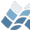NV5 GEOSPATIAL SOFTWAREARRAY_TOTAL

ARRAY_TOTAL

## Purpose

This function does more flexible array integration than IDL's total.

Array

## Calling Sequence

Result = array_total( Data [, Instruct] )

## Inputs

Data: A numerical array of data to be processed, of any dimension.
Instruct: An array of type string containing processing instructions,
performed sequentially in the given order. Each instruction is
of the form 'INSTRUCT_TYPE=INSTRUCT_DIM'. Possible values for
INSTRUCT_TYPE are:
'total': calculate the total along the INSTRUCT_DIMdimension(s)
'mean': calculate the mean along the INSTRUCT_DIMdimension(s).
INSTRUCT_DIM is a sequence of dimension indices separated by
commas. These are the same as for IDL's total function, i.e.
the left-most dimension is '1'. If multiple dimensions are
listed in a single instruction, then those dimensions are
treated as one and the operation is performed simultaneously on
all of them. So 'mean=1,2' will average over dimensions 1 and
2 simulataneously, while ['mean=1','mean=2'] will first average
over dimension 1 and average then over dimension 2.
FRAC_COVERAGE_THRESH, NAN, REFORM, WEIGHT

## Keyword Parameters

COVERAGE: Returns an array of the same dimensions of Result containing
the fractional coverage of values in data, relative to the
total possible (i.e. the (weighted) fraction of values that are
not NaN), involved in the calculation of the final instruction
in Instruct which produces Result.
FRAC_COVERAGE_THRESH: The minimum fraction of (weighted) data used in
each operation that must be defined (i.e. not NaN) in order to
proceed with the calculation. If the criterion is not met
then a NaN is returned from that calculation. The default is 0.
NAN: If set then NaNs (not-a-number values) are ignored in the
calculations. The default is not set.
REFORM: If set then the reform function is performed on Result,
COVERAGE, DATA_MEAN, and MEAN COVERAGE to remove all 1-element
dimensions. The default is for these output to maintain the
original number of dimensions, with some dimensions reduced to
1-element size according to Instruct and REFERENCE.
WEIGHT: An array of the same size as DATA containing relative
weightings for the corresponding elements in DATA. All
calculations producing Result, COVERAGE, DATA_MEAN, and
MEAN_COVERAGE take account of these weightings. If not set
then identical weightings are assumed for all elements.

## Outputs

Result: Returns the processed version of Data. This is of the same
size as Data except that the dimensions with instructions
specified in Instruct have only one element. If REFORM is set
then these 1-element dimensions are removed.
COVERAGE

## Modification History

Written by: Daithi A. Stone (stoned@atm.ox.ac.uk), 2008-03-11,